# Chapter 3 Data Visualisation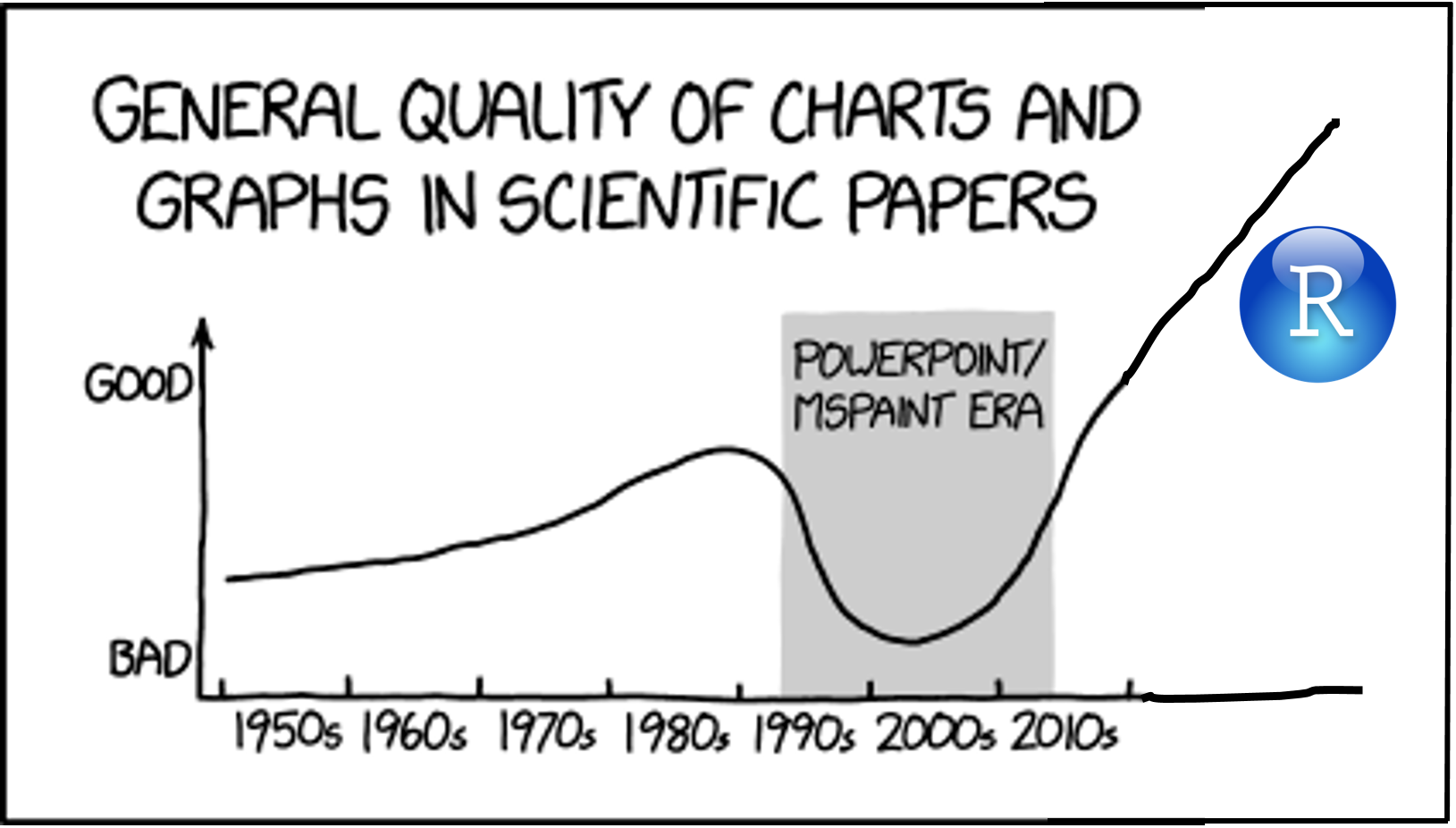Take the quiz to see if you need to review this chapter.

## 3.1 Learning Objectives

### 3.1.1 Basic

1. Understand what types of graphs are best for different types of data
• 1 discrete
• 1 continuous
• 2 discrete
• 2 continuous
• 1 discrete, 1 continuous
• 3 continuous
2. Create common types of graphs with ggplot2
3. Set custom labels and colours
4. Represent factorial designs with different colours or facets
5. Save plots as an image file

### 3.1.2 Intermediate

1. Superimpose different types of graphs
3. Deal with overlapping data
4. Create less common types of graphs

1. Arrange plots in a grid using `cowplot`
2. Adjust axes (e.g., flip coordinates, set axis limits)
3. Change the theme
4. Create interactive graphs with `plotly`

## 3.2 Resources

Stub for this lesson

## 3.3 Setup

``````# libraries needed for these graphs
library(tidyverse)
library(plotly)
library(cowplot)
set.seed(30250) # makes sure random numbers are reproducible``````

## 3.4 Common Variable Combinations

Continuous variables are properties you can measure, like height. Discrete (or categorical) variables are things you can count, like the number of pets you have. Categorical variables can be nominal, where the categories don't really have an order, like cats, dogs and ferrets (even though ferrets are obviously best). They can also be ordinal, where there is a clear order, but the distance between the categories isn't something you could exactly equate, like points on a Likert rating scale.

Different types of visualisations are good for different types of variables.

Before you read ahead, come up with an example of each type of variable combination and sketch the types of graphs that would best display these data.

• 1 discrete
• 1 continuous
• 2 discrete
• 2 continuous
• 1 discrete, 1 continuous
• 3 continuous

### 3.4.1 Data

The code below creates some data frames with different types of data. We'll learn how to simulate data like this in the Probability & Simulation chapter, but for now just run the code chunk below.

• `pets` has a column with pet type
• `pet_happy` has `happiness` and `age` for 500 dog owners and 500 cat owners
• `x_vs_y` has two correlated continuous variables (`x` and `y`)
• `overlap` has two correlated ordinal variables and 1000 observations so there is a lot of overlap
• `overplot` has two correlated continuous variables and 10000 observations

First, think about what kinds of graphs are best for representing these different types of data.

``````pets <- tibble(
pet = sample(
c("dog", "cat", "ferret", "bird", "fish"),
100,
TRUE,
c(0.45, 0.40, 0.05, 0.05, 0.05)
)
)

pet_happy <- tibble(
pet = rep(c("dog", "cat"), each = 500),
happiness = c(rnorm(500, 55, 10), rnorm(500, 45, 10)),
age = rpois(1000, 3) + 20
)

x_vs_y <- tibble(
x = rnorm(100),
y = x + rnorm(100, 0, 0.5)
)

overlap <- tibble(
x = rbinom(1000, 10, 0.5),
y = x + rbinom(1000, 20, 0.5)
)

overplot <- tibble(
x = rnorm(10000),
y = x + rnorm(10000, 0, 0.5)
)``````

## 3.5 Basic Plots

### 3.5.1 Bar plot

Bar plots are good for categorical data where you want to represent the count.

``````ggplot(pets, aes(pet)) +
geom_bar()``````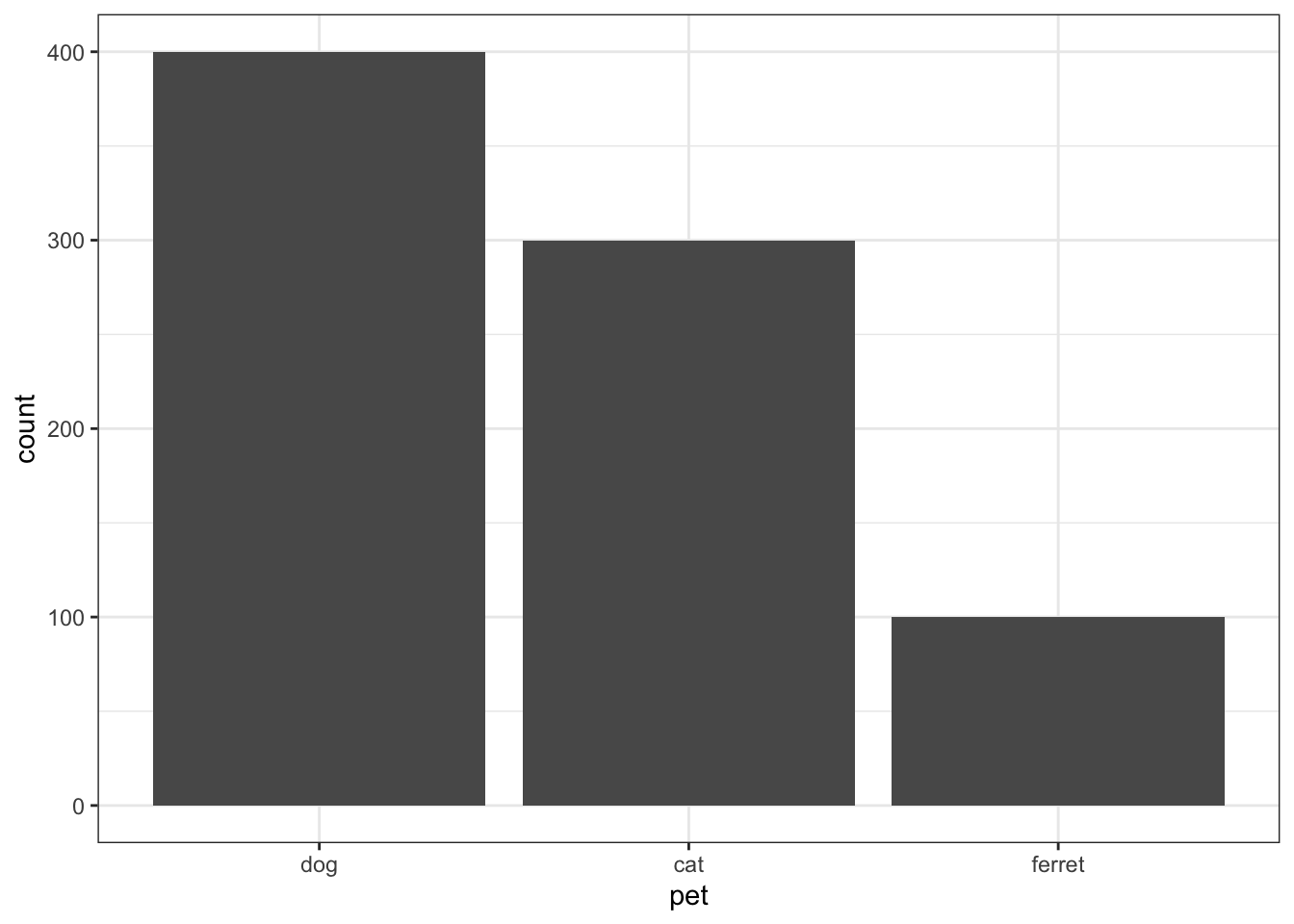Figure 3.1: Bar plot

### 3.5.2 Density plot

Density plots are good for one continuous variable, but only if you have a fairly large number of observations.

``````ggplot(pet_happy, aes(happiness)) +
geom_density()``````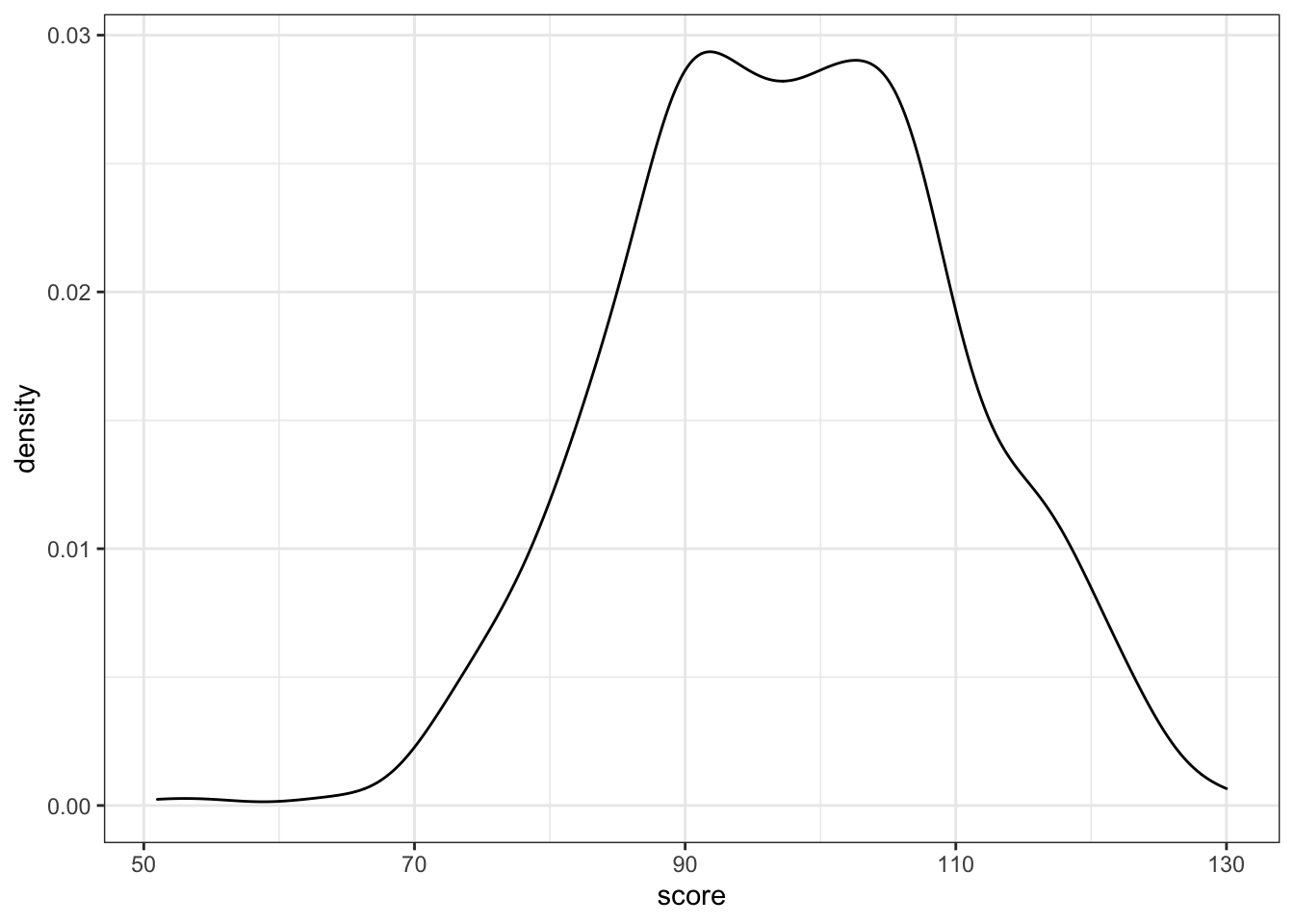Figure 3.2: Density plot

You can represent subsets of a variable by assigning the category variable to the argument `group`, `fill`, or `color`.

``````ggplot(pet_happy, aes(happiness, fill = pet)) +
geom_density(alpha = 0.5)``````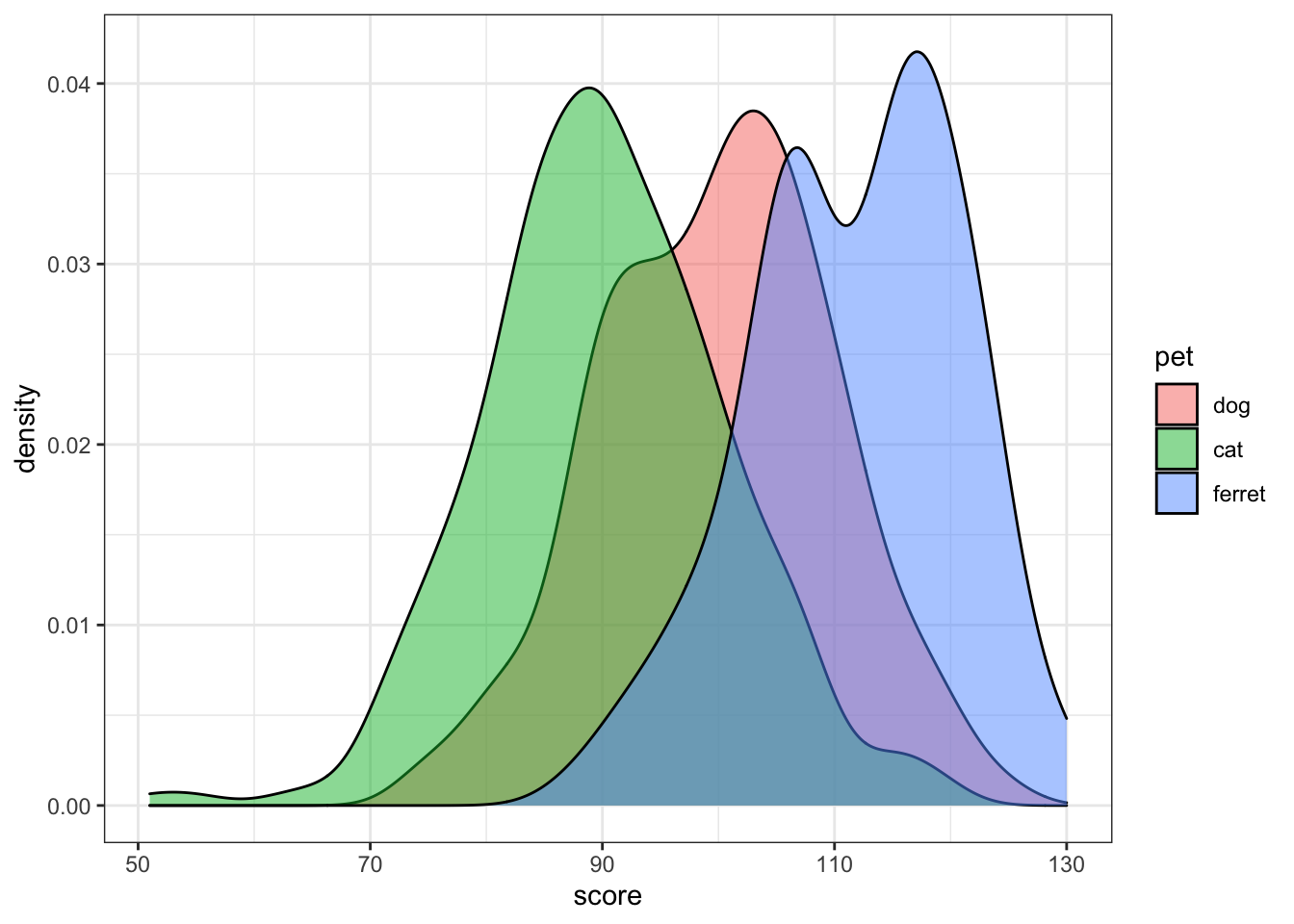Figure 3.3: Grouped density plot

Try changing the `alpha` argument to figure out what it does.

### 3.5.3 Frequency Polygons

If you don't want smoothed distributions, try `geom_freqpoly()`.

``````ggplot(pet_happy, aes(happiness, color = pet)) +
geom_freqpoly(binwidth = 1)``````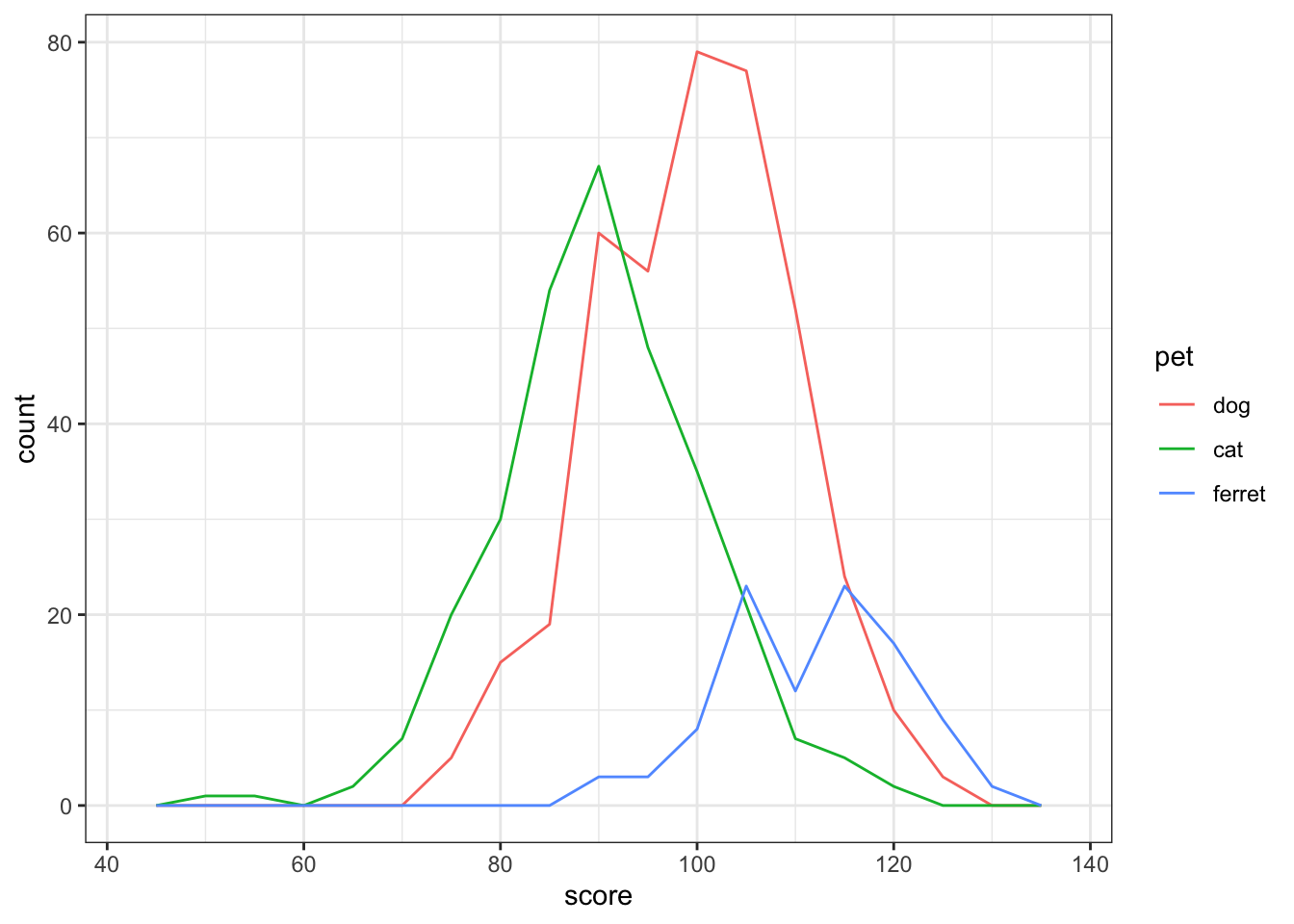Figure 3.4: Frequency ploygon plot

Try changing the `binwidth` argument to 5 and 0.1. How do you figure out the right value?

### 3.5.4 Histogram

Histograms are also good for one continuous variable, and work well if you don't have many observations. Set the `binwidth` to control how wide each bar is.

``````ggplot(pet_happy, aes(happiness)) +
geom_histogram(binwidth = 1, fill = "white", color = "black")``````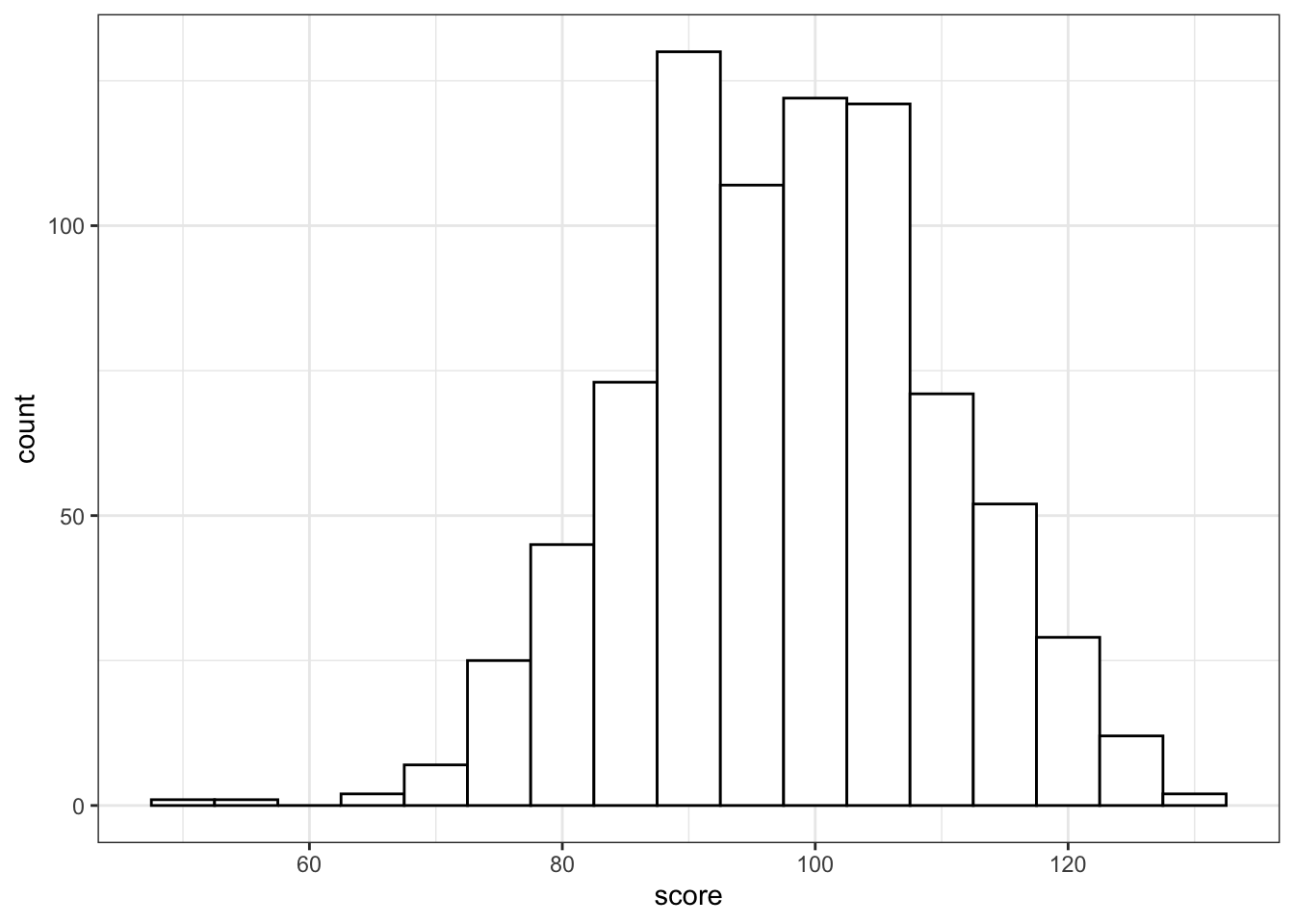Figure 3.5: Histogram

Histograms in ggplot look pretty bad unless you set the `fill` and `color`.

If you show grouped histograms, you also probably want to change the default `position` argument.

``````ggplot(pet_happy, aes(happiness, fill=pet)) +
geom_histogram(binwidth = 1, alpha = 0.5, position = "dodge")``````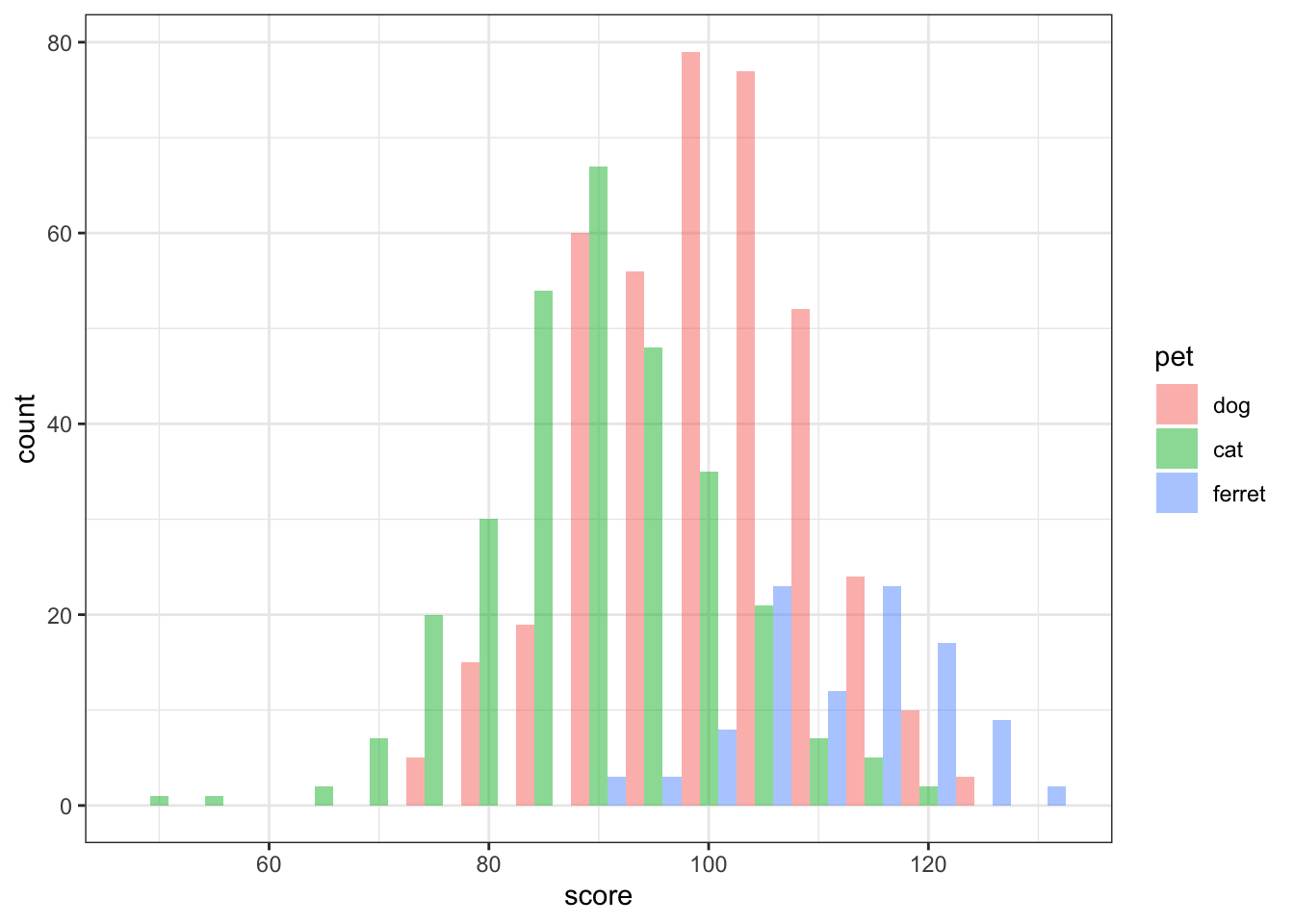Figure 3.6: Grouped Histogram

Try changing the `position` argument to "identity", "fill", "dodge", or "stack".

### 3.5.5 Column plot

Column plots are the worst way to represent grouped continuous data, but also one of the most common.

To make column plots with error bars, you first need to calculate the means, error bar uper limits (`ymax`) and error bar lower limits (`ymin`) for each category. You'll learn more about how to use the code below in the next two lessons.

``````# calculate mean and SD for each pet
avg_pet_happy <- pet_happy %>%
group_by(pet) %>%
summarise(
mean = mean(happiness),
sd = sd(happiness)
)

ggplot(avg_pet_happy, aes(pet, mean, fill=pet)) +
geom_col(alpha = 0.5) +
geom_errorbar(aes(ymin = mean - sd, ymax = mean + sd), width = 0.25) +
geom_hline(yintercept = 40)``````Figure 3.7: Column plot

What do you think `geom_hline()` does?

### 3.5.6 Boxplot

Boxplots are great for representing the distribution of grouped continuous variables. They fix most of the problems with using barplots for continuous data.

``````ggplot(pet_happy, aes(pet, happiness, fill=pet)) +
geom_boxplot(alpha = 0.5)``````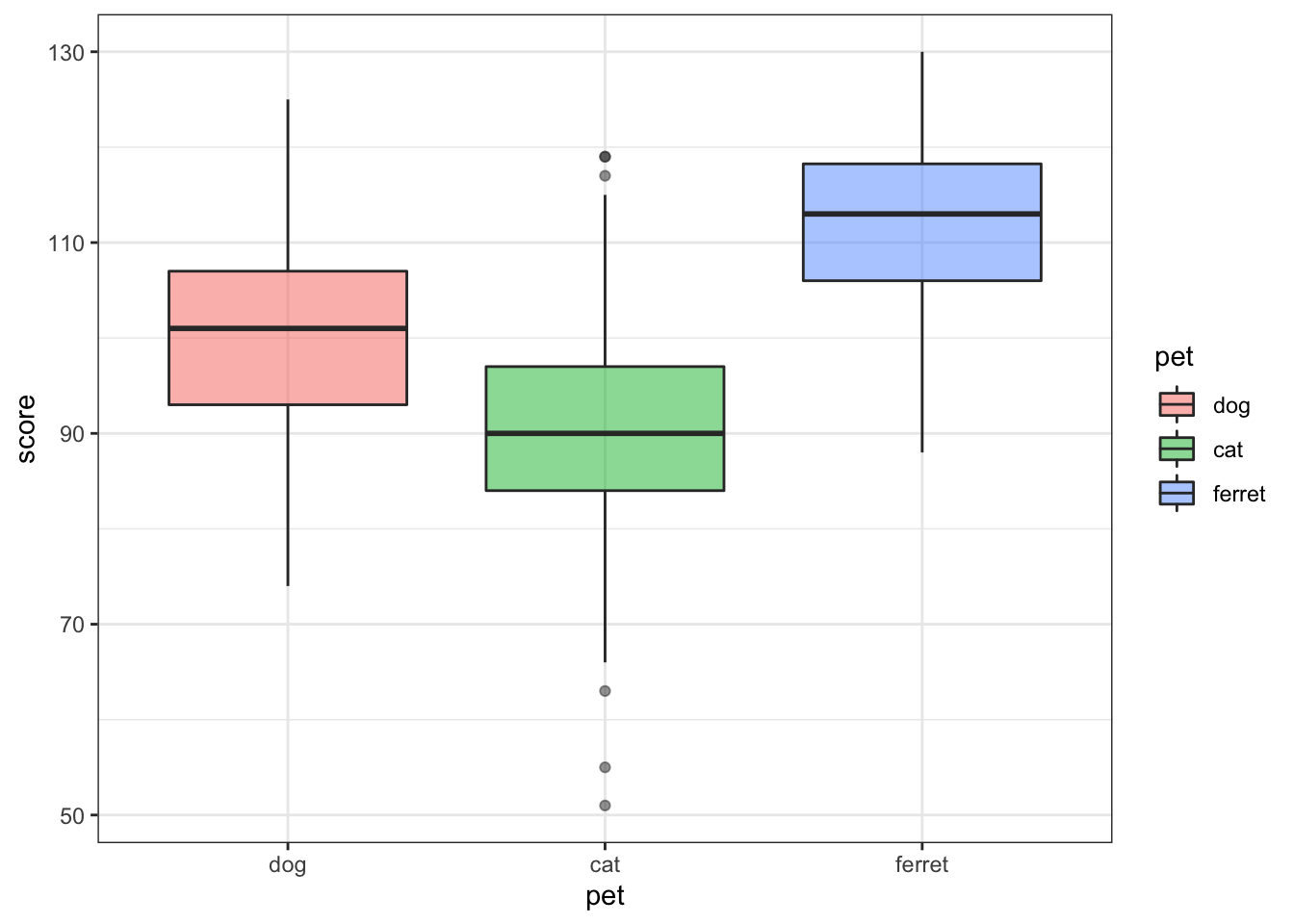Figure 3.8: Box plot

### 3.5.7 Violin plot

Violin pots are like sideways, mirrored density plots. They give even more information than a boxplot about distribution and are especially useful when you have non-normal distributions.

``````ggplot(pet_happy, aes(pet, happiness, fill=pet)) +
geom_violin(
trim = FALSE,
draw_quantiles = c(0.25, 0.5, 0.75),
alpha = 0.5
)``````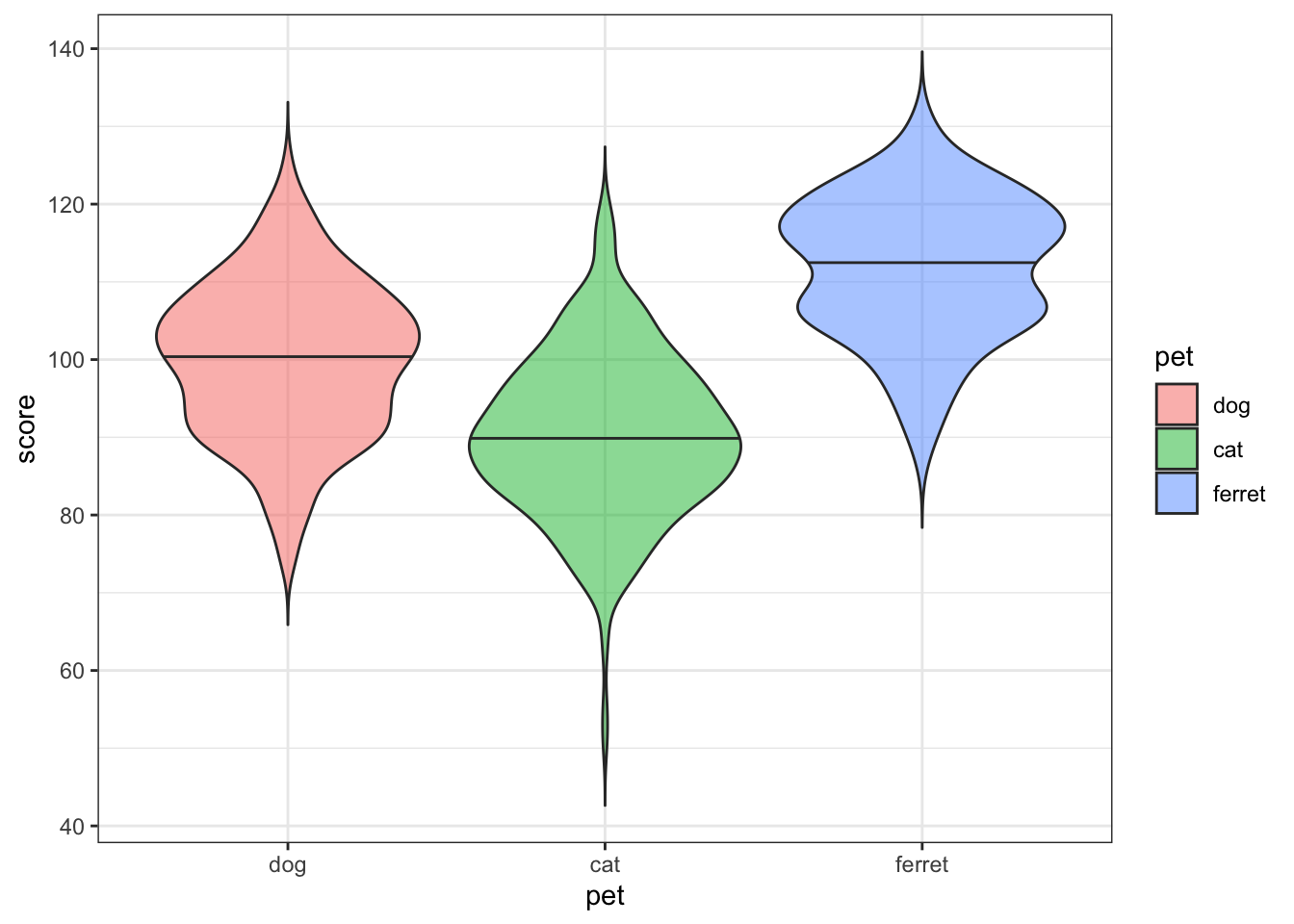Figure 3.9: Violin plot

Try changing the numbers in the `draw_quantiles` argument.

### 3.5.8 Scatter plot

Scatter plots are a good way to represent the relationship between two continuous variables.

``````ggplot(x_vs_y, aes(x, y)) +
geom_point()``````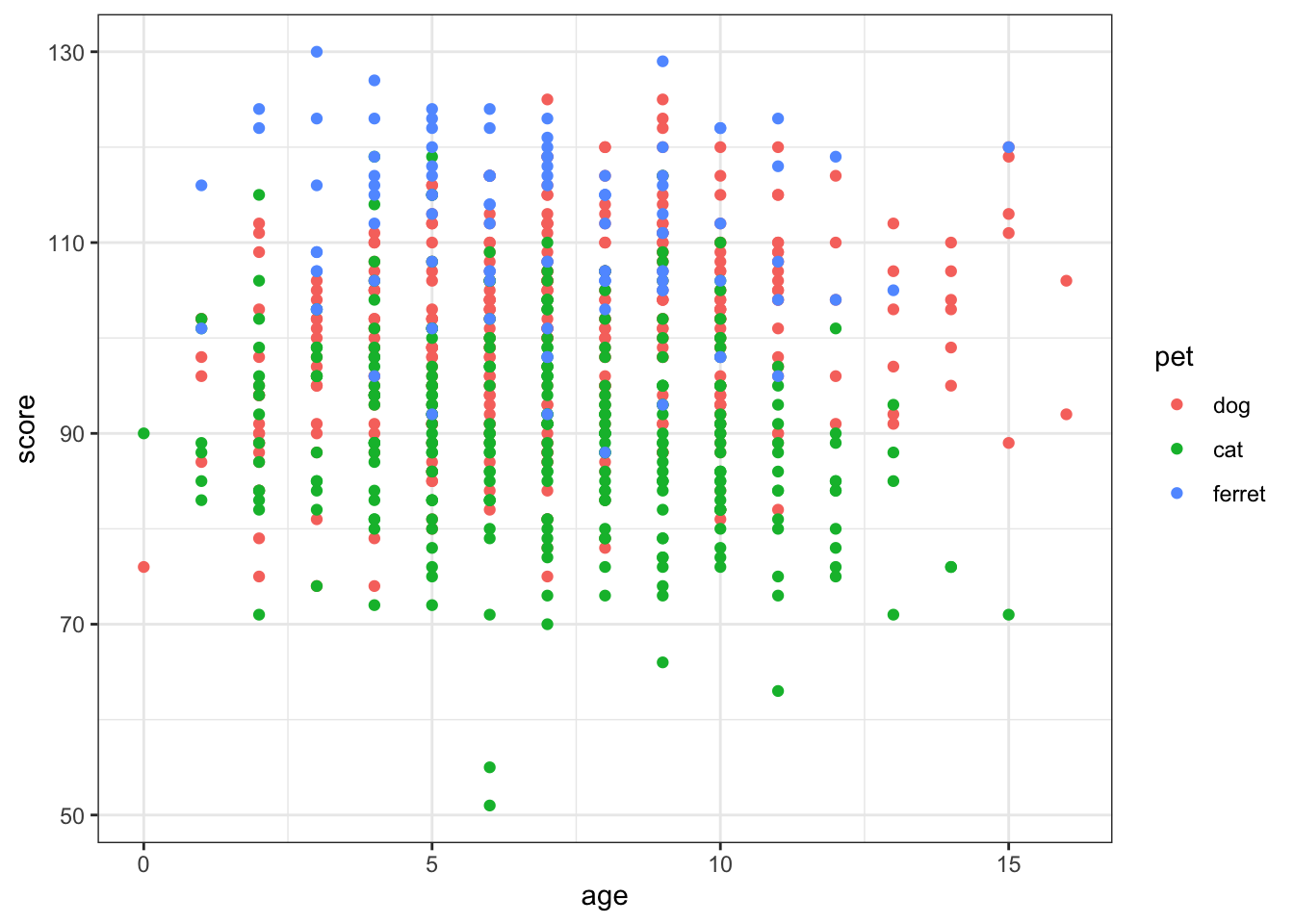Figure 3.10: Scatter plot using geom_point()

### 3.5.9 Line graph

You often want to represent the relationship as a single line.

``````ggplot(x_vs_y, aes(x, y)) +
geom_smooth(method="lm")``````Figure 3.11: Line plot using geom_smooth()

## 3.6 Customisation

### 3.6.1 Labels

You can set custom titles and axis labels in a few different ways.

``````ggplot(x_vs_y, aes(x, y)) +
geom_smooth(method="lm") +
ggtitle("My Plot Title") +
xlab("The X Variable") +
ylab("The Y Variable")``````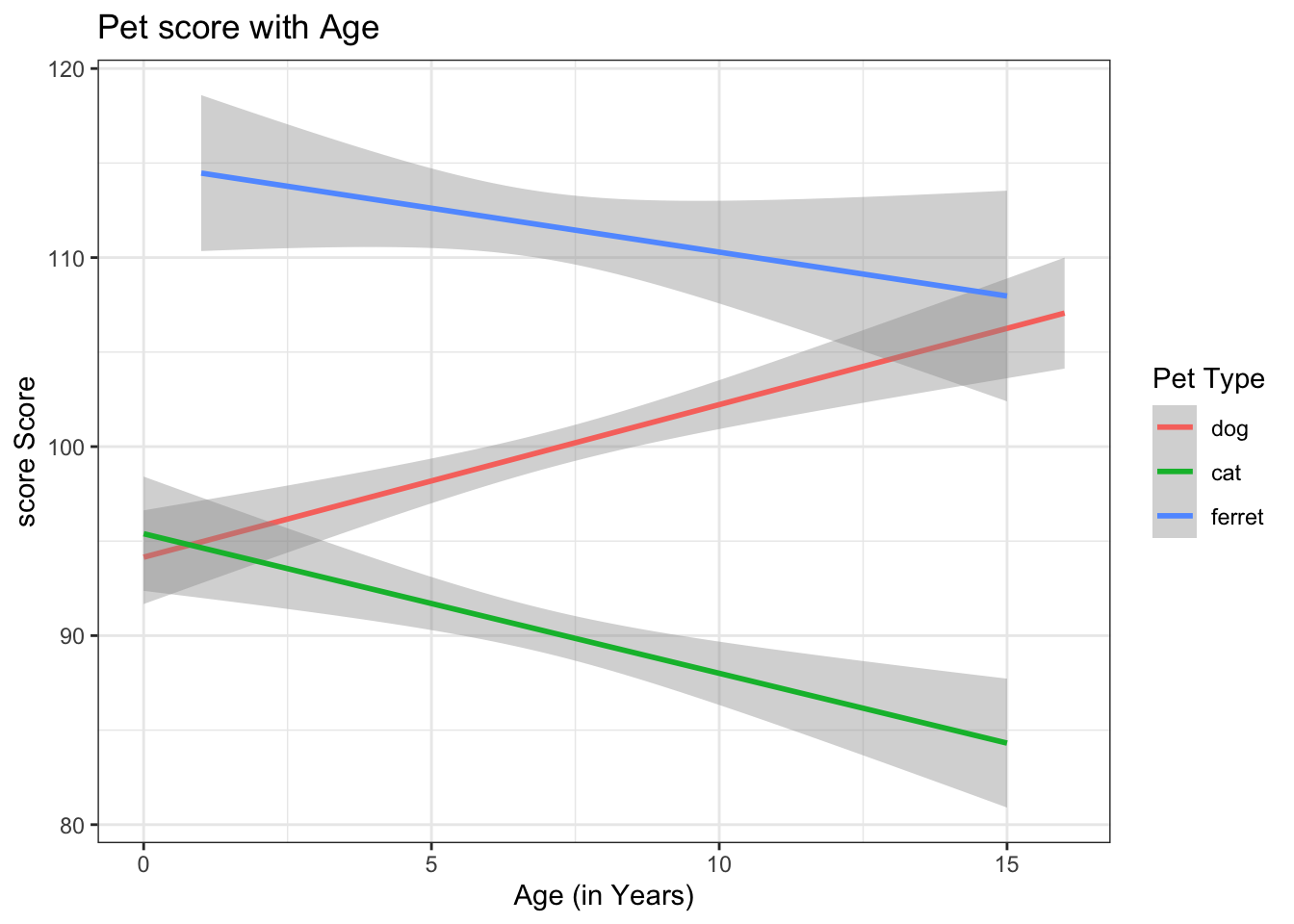Figure 3.12: Set custom labels with ggtitle(), xlab() and ylab()

``````ggplot(x_vs_y, aes(x, y)) +
geom_smooth(method="lm") +
labs(title = "My Plot Title",
x = "The X Variable",
y = "The Y Variable")``````Figure 3.13: Set custom labels with labs()

### 3.6.2 Colours

You can set custom values for colour and fill using functions like `scale_colour_manual()` and `scale_fill_manual()`. The Colours chapter in Cookbook for R has many more ways to customise colour.

``````ggplot(pet_happy, aes(pet, happiness, colour = pet, fill = pet)) +
geom_violin() +
scale_color_manual(values = c("darkgreen", "dodgerblue")) +
scale_fill_manual(values = c("#CCFFCC", "#BBDDFF"))``````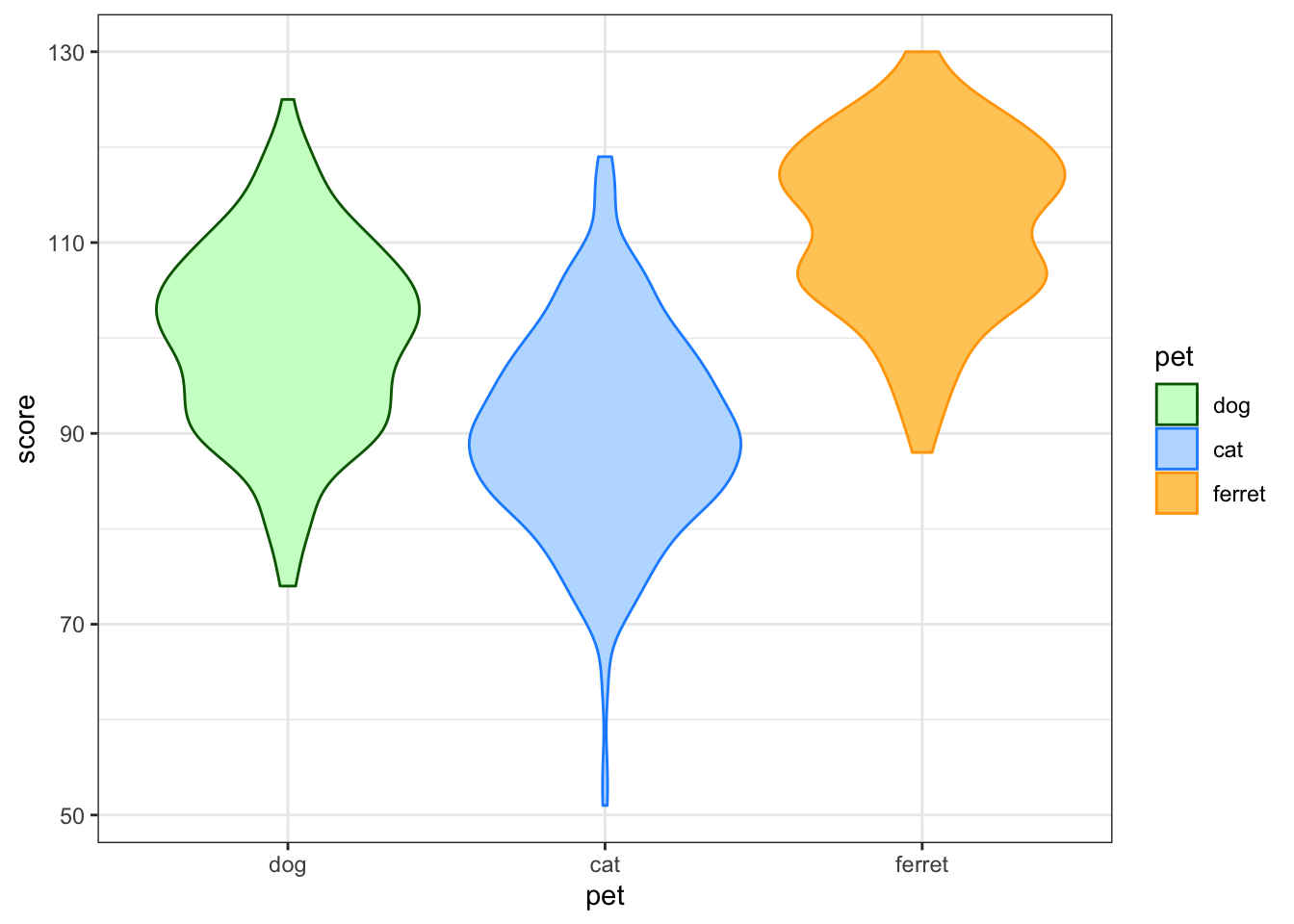Figure 3.14: Set custom colour

### 3.6.3 Save as File

You can save a ggplot using `ggsave()`. It saves the last ggplot you made, by default, but you can specify which plot you want to save if you assigned that plot to a variable.

You can set the `width` and `height` of your plot. The default units are inches, but you can change the `units` argument to "in", "cm", or "mm".

``````box <- ggplot(pet_happy, aes(pet, happiness, fill=pet)) +
geom_boxplot(alpha = 0.5)

violin <- ggplot(pet_happy, aes(pet, happiness, fill=pet)) +
geom_violin(alpha = 0.5)

ggsave("demog_violin_plot.png", width = 5, height = 7)

ggsave("demog_box_plot.jpg", plot = box, width = 5, height = 7)``````

## 3.7 Combination Plots

### 3.7.1 Violinbox plot

To demonstrate the use of `facet_grid()` for factorial designs, we create a new column called `agegroup` to split the data into participants older than the meadian age or younger than the median age. New factors will display in alphabetical order, so we can use the `factor()` function to set the levels in the order we want.

``````pet_happy %>%
mutate(agegroup = ifelse(age<median(age), "Younger", "Older"),
agegroup = factor(agegroup, levels = c("Younger", "Older"))) %>%
ggplot(aes(pet, happiness, fill=pet)) +
geom_violin(trim = FALSE, alpha=0.5, show.legend = FALSE) +
geom_boxplot(width = 0.25, fill="white") +
facet_grid(.~agegroup) +
scale_fill_manual(values = c("orange", "green"))``````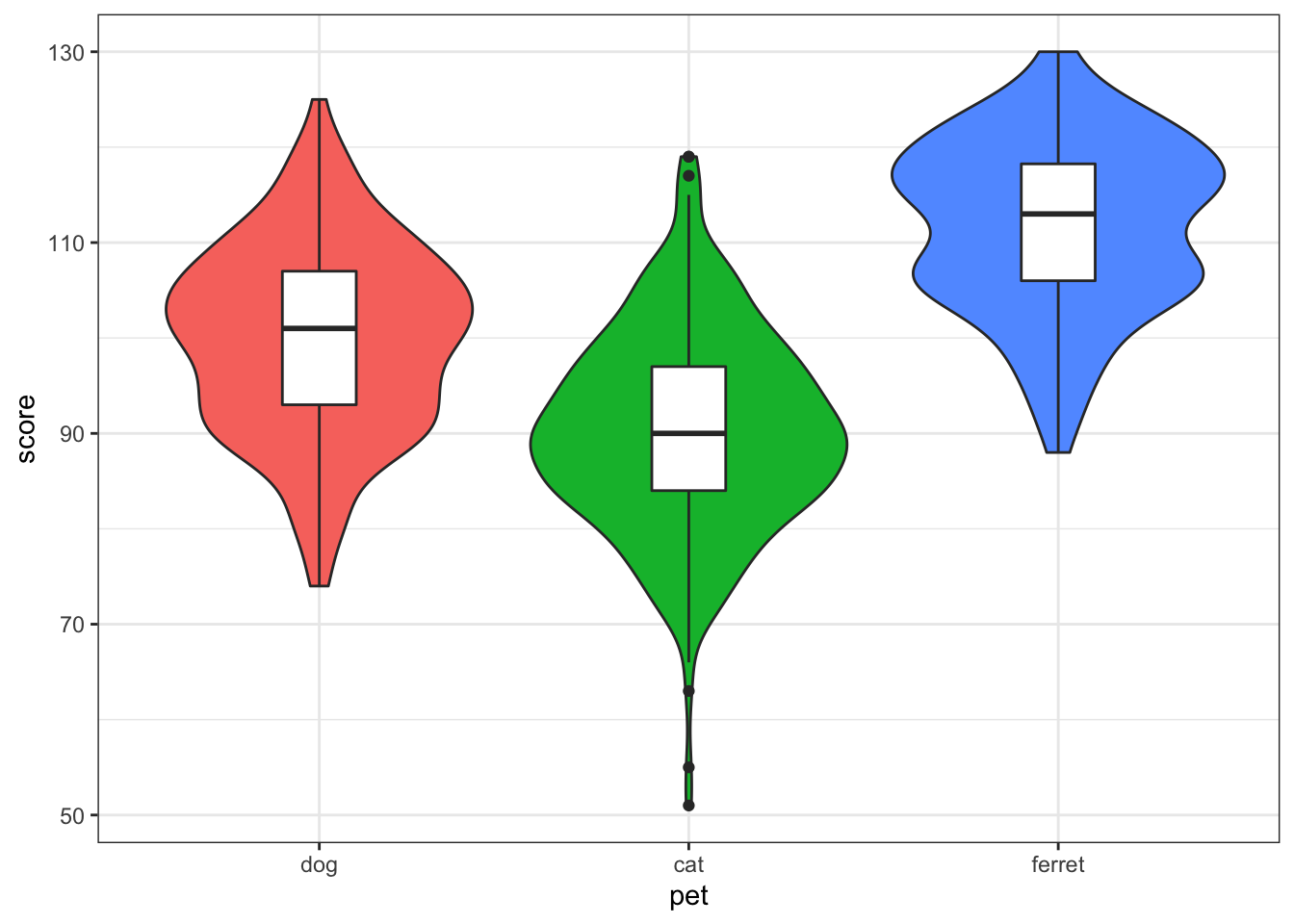Figure 3.15: Violin-box plot

Set the `show.legend` argument to `FALSE` to hide the legend. We do this here because the x-axis already labels the pet types.

### 3.7.2 Violin-point-range plot

You can use `stat_summary()` to superimpose a point-range plot showning the mean ± 1 SD. You'll learn how to write your own functions in the lesson on Iteration and Functions.

``````ggplot(pet_happy, aes(pet, happiness, fill=pet)) +
geom_violin(
trim = FALSE,
alpha = 0.5
) +
stat_summary(
fun.y = mean,
fun.ymax = function(x) {mean(x) + sd(x)},
fun.ymin = function(x) {mean(x) - sd(x)},
geom="pointrange"
)``````Figure 3.16: Point-range plot using stat_summary()

### 3.7.3 Violin-jitter plot

If you don't have a lot of data points, it's good to represent them individually. You can use `geom_jitter` to do this.

``````pet_happy %>%
sample_n(50) %>%  # choose 50 random observations from the dataset
ggplot(aes(pet, happiness, fill=pet)) +
geom_violin(
trim = FALSE,
draw_quantiles = c(0.25, 0.5, 0.75),
alpha = 0.5
) +
geom_jitter(
width = 0.15, # points spread out over 15% of available width
height = 0, # do not move position on the y-axis
alpha = 0.5,
size = 3
)``````Figure 3.17: Violin-jitter plot

### 3.7.4 Scatter-line graph

If your graph isn't too complicated, it's good to also show the individual data points behind the line.

``````ggplot(x_vs_y, aes(x, y)) +
geom_point(alpha = 0.25) +
geom_smooth(method="lm")``````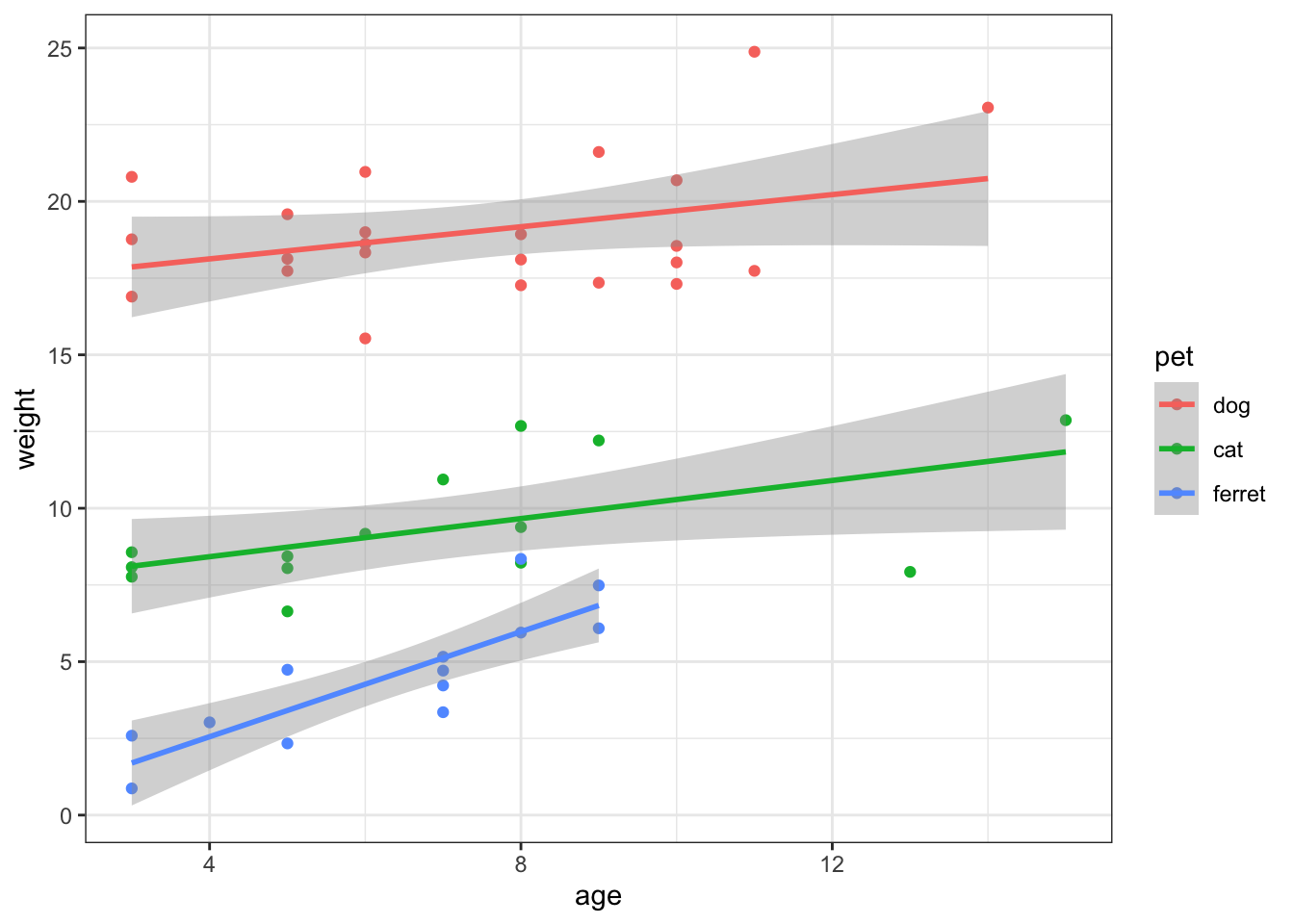Figure 3.18: Scatter-line plot

### 3.7.5 Grid of plots

You can use the `cowplot` package to easily make grids of different graphs. First, you have to assign each plot a name. Then you list all the plots as the first arguments of `plot_grid()` and provide a list of labels.

``````my_hist <- ggplot(pet_happy, aes(happiness, fill=pet)) +
geom_histogram(
binwidth = 1,
alpha = 0.5,
position = "dodge",
show.legend = FALSE
)

my_violin <- ggplot(pet_happy, aes(pet, happiness, fill=pet)) +
geom_violin(
trim = FALSE,
draw_quantiles = c(0.5),
alpha = 0.5,
show.legend = FALSE
)

my_box <- ggplot(pet_happy, aes(pet, happiness, fill=pet)) +
geom_boxplot(alpha=0.5, show.legend = FALSE)

my_density <- ggplot(pet_happy, aes(happiness, fill=pet)) +
geom_density(alpha=0.5, show.legend = FALSE)

my_bar <- pet_happy %>%
group_by(pet) %>%
summarise(
mean = mean(happiness),
sd = sd(happiness)
) %>%
ggplot(aes(pet, mean, fill=pet)) +
geom_bar(stat="identity", alpha = 0.5,
color = "black", show.legend = FALSE) +
geom_errorbar(aes(ymin = mean - sd, ymax = mean + sd), width = 0.25)

plot_grid(
my_violin,
my_box,
my_density,
my_bar,
labels = c("A", "B", "C", "D")
)``````Figure 3.19: Grid of plots using cowplot

## 3.8 Overlapping Discrete Data

### 3.8.1 Reducing Opacity

You can deal with overlapping data points (very common if you're using Likert scales) by reducing the opacity of the points. You need to use trial and error to adjust these so they look right.

``````ggplot(overlap, aes(x, y)) +
geom_point(size = 5, alpha = .05) +
geom_smooth(method="lm")``````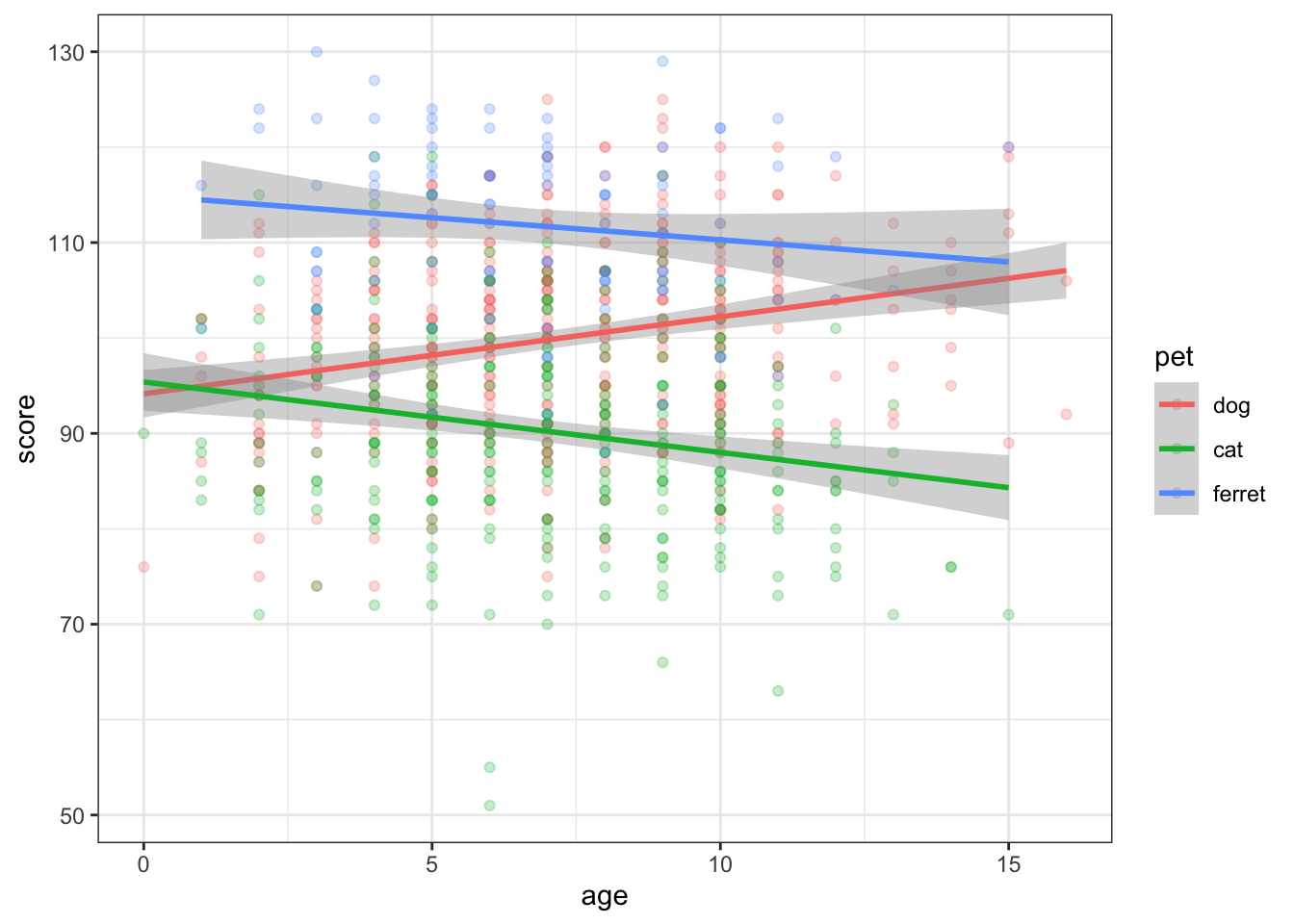Figure 3.20: Deal with overlapping data using transparency

### 3.8.2 Proportional Dot Plots

Or you can set the size of the dot proportional to the number of overlapping observations using `geom_count()`.

``````overlap %>%
ggplot(aes(x, y)) +
geom_count(color = "#663399")``````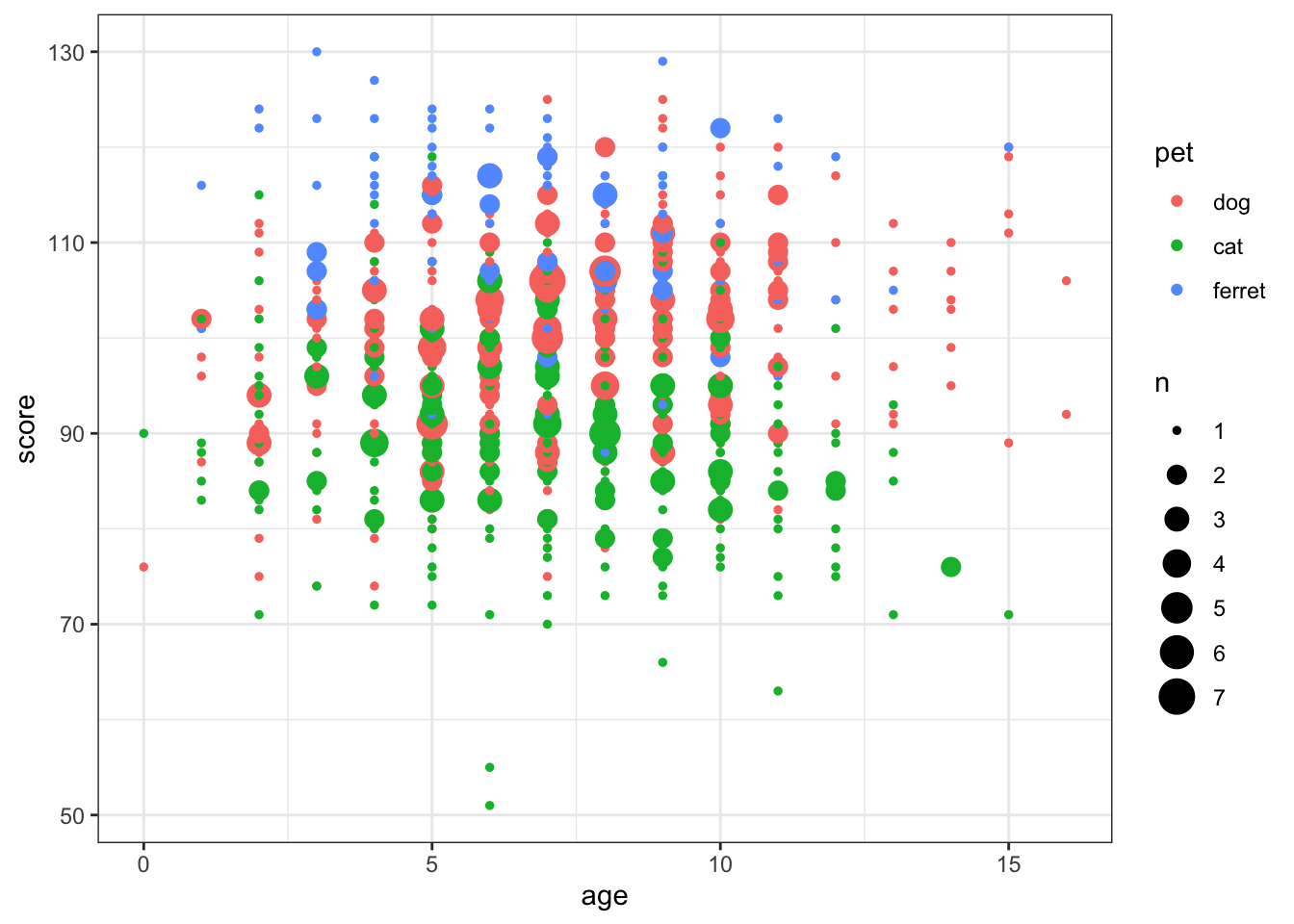Figure 3.21: Deal with overlapping data using geom_count()

Alternatively, you can transform your data to create a count column and use the count to set the dot colour.

``````overlap %>%
group_by(x, y) %>%
summarise(count = n()) %>%
ggplot(aes(x, y, color=count)) +
geom_point(size = 5) +
scale_color_viridis_c()``````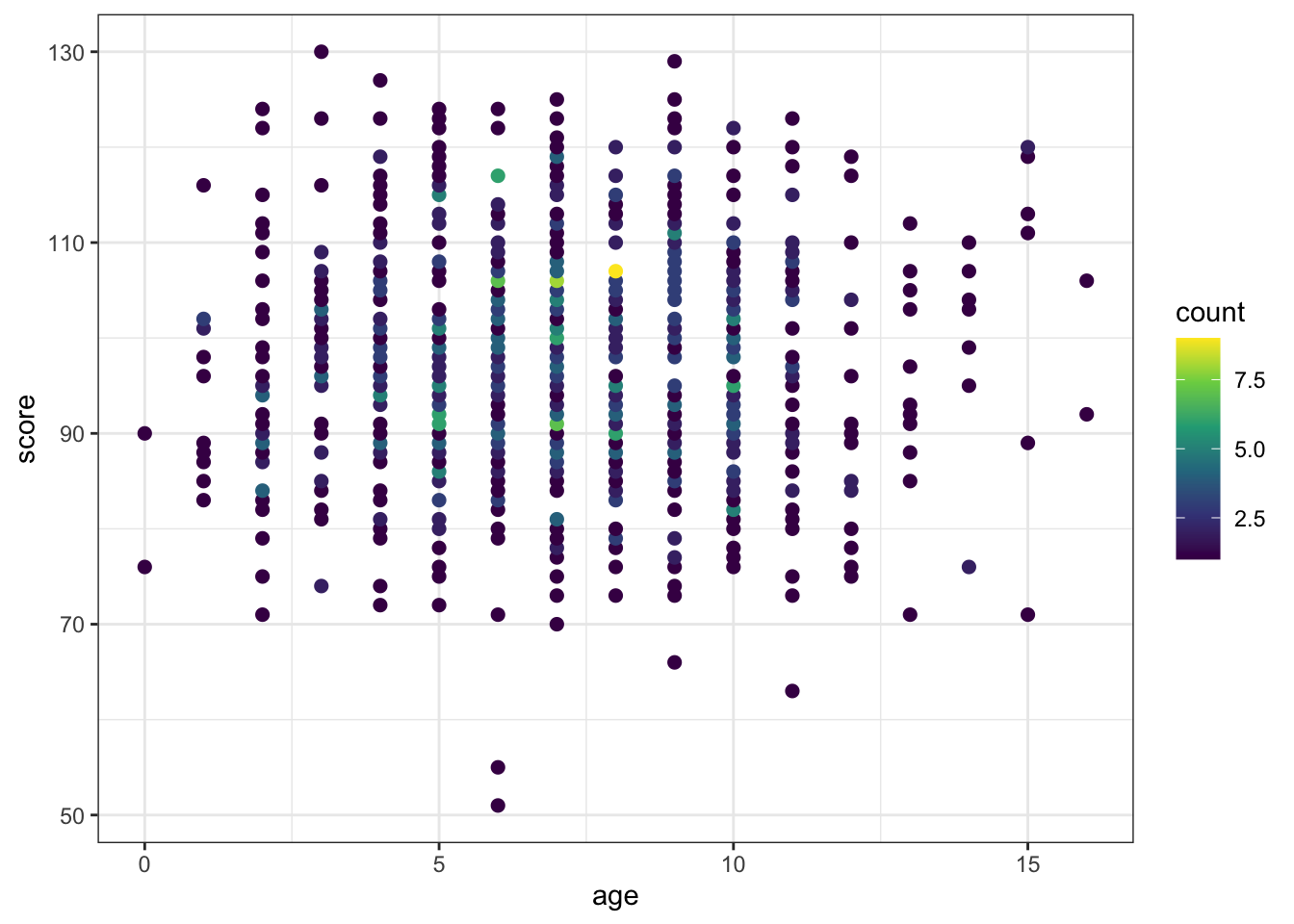Figure 3.22: Deal with overlapping data using dot colour

The viridis package changes the colour themes to be easier to read by people with colourblindness and to print better in greyscale. Viridis is built into `ggplot2` since v3.0.0. It uses `scale_colour_viridis_c()` and `scale_fill_viridis_c()` for continuous variables and `scale_colour_viridis_d()` and `scale_fill_viridis_d()` for discrete variables.

## 3.9 Overlapping Continuous Data

Even if the variables are continuous, overplotting might obscure any relationships if you have lots of data.

``````overplot %>%
ggplot(aes(x, y)) +
geom_point()``````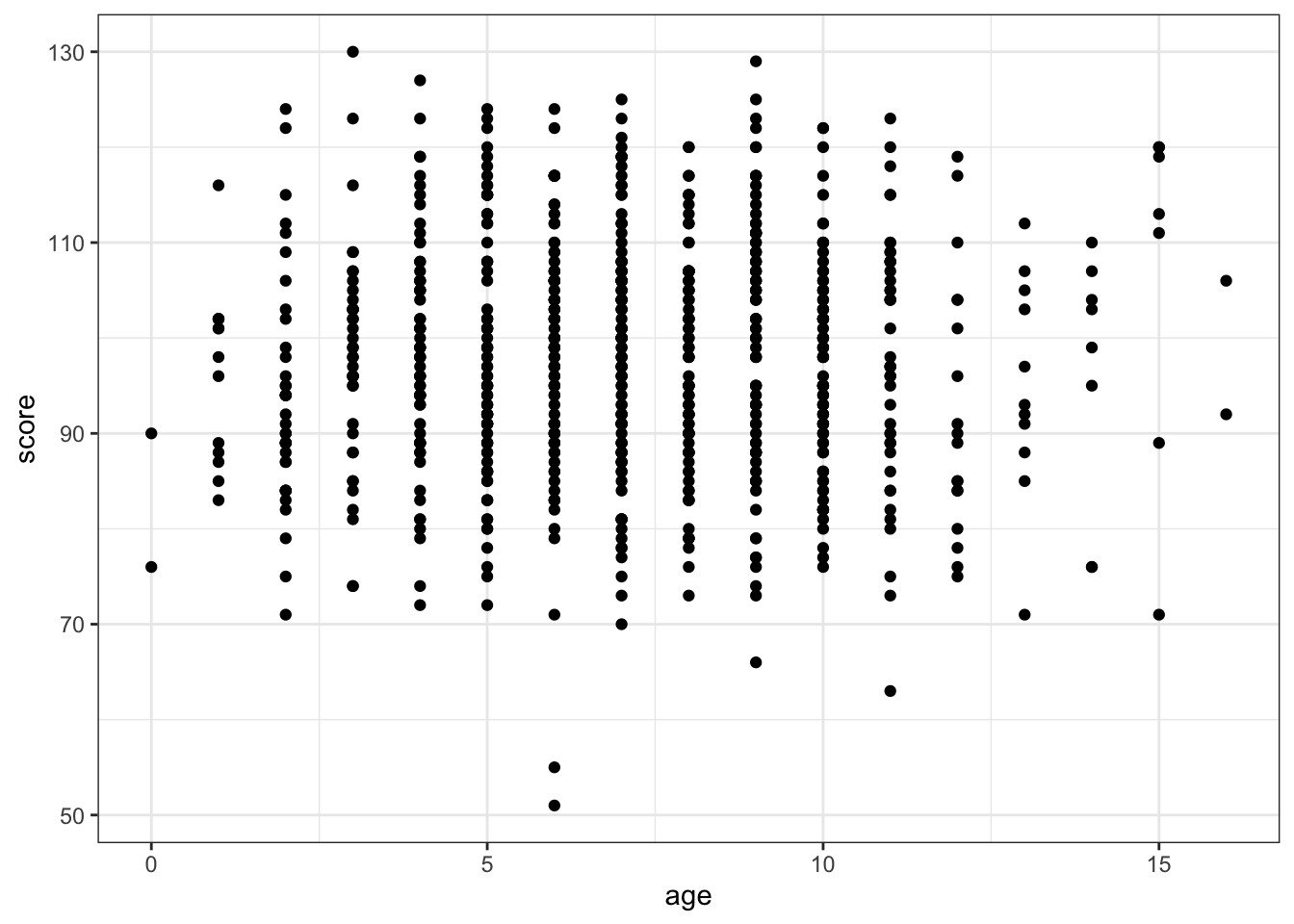Figure 3.23: Overplotted data

### 3.9.1 2D Density Plot

Use `geom_density2d()` to create a contour map.

``````overplot %>%
ggplot(aes(x, y)) +
geom_density2d()``````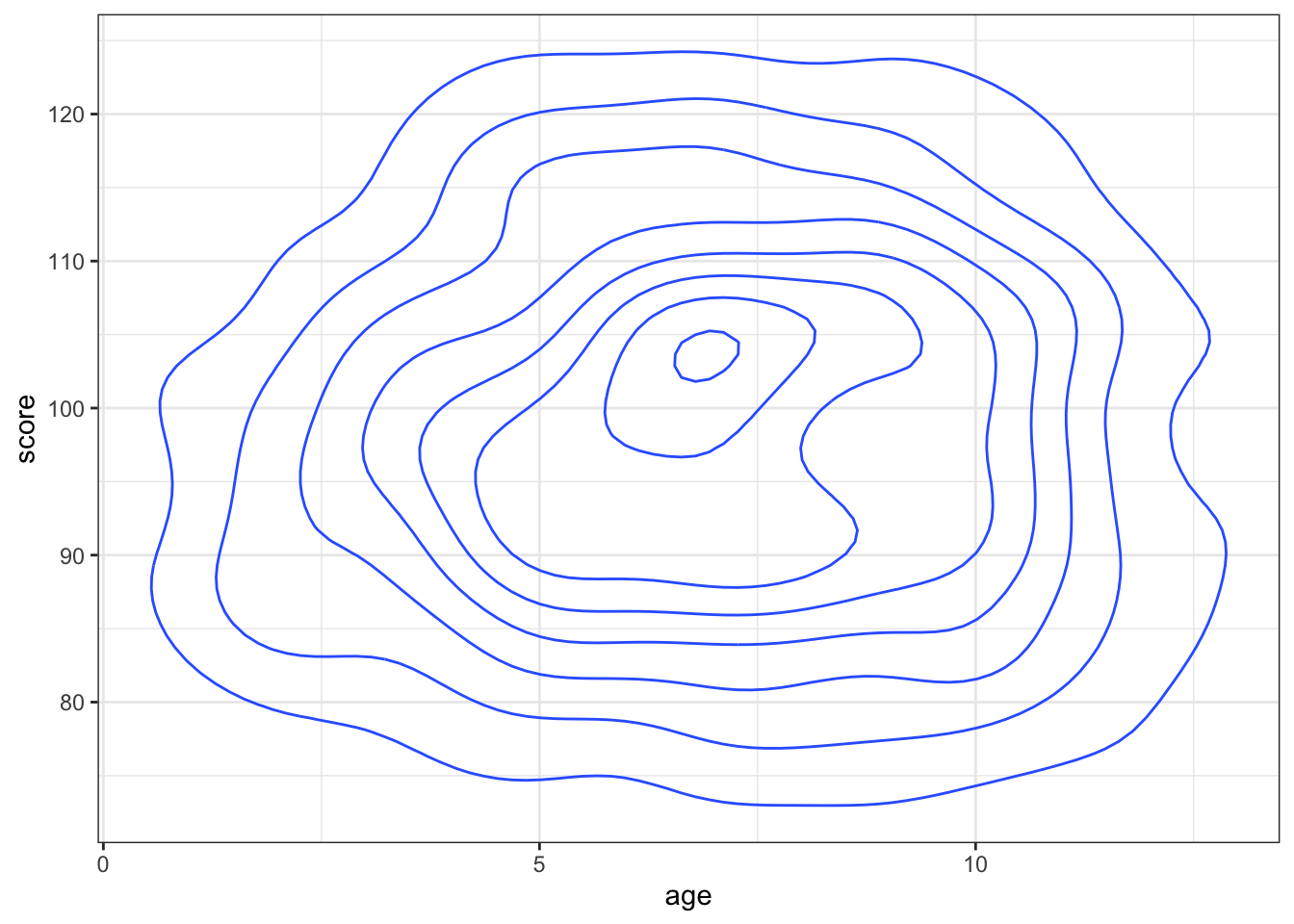Figure 3.24: Contour map with geom_density2d()

You can use `stat_density_2d(aes(fill = ..level..), geom = "polygon")` to create a heatmap-style density plot.

``````overplot %>%
ggplot(aes(x, y)) +
stat_density_2d(aes(fill = ..level..), geom = "polygon") +
scale_fill_viridis_c()``````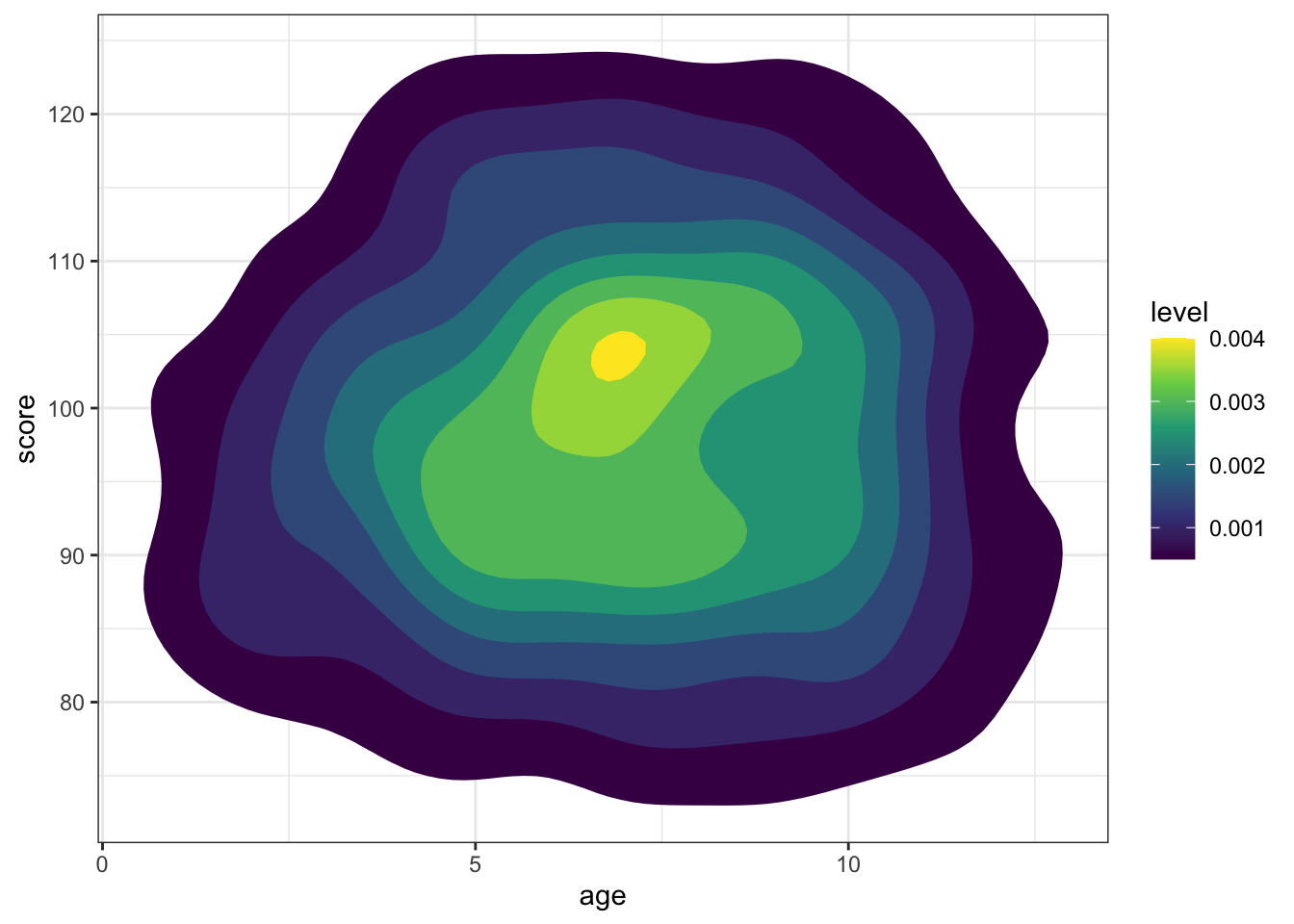Figure 3.25: Heatmap-density plot

### 3.9.2 2D Histogram

Use `geom_bin2d()` to create a rectangular heatmap of bin counts. Set the `binwidth` to the x and y dimensions to capture in each box.

``````overplot %>%
ggplot(aes(x, y)) +
geom_bin2d(binwidth = c(1,1))``````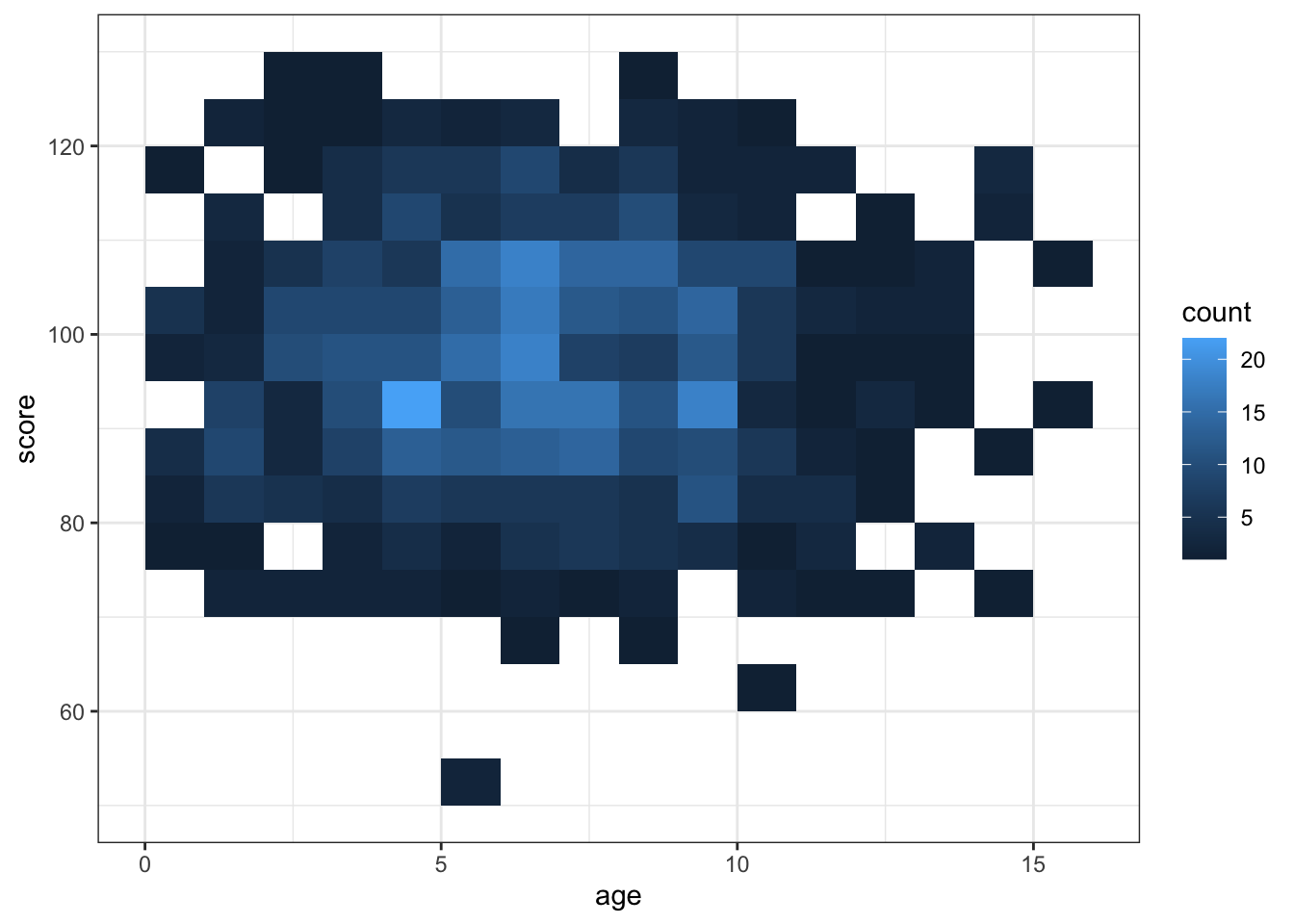Figure 3.26: Heatmap of bin counts

### 3.9.3 Hexagonal Heatmap

Use `geomhex()` to create a hexagonal heatmap of bin counts. Adjust the `binwidth`, `xlim()`, `ylim()` and/or the figure dimensions to make the hexagons more or less stretched.

``````overplot %>%
ggplot(aes(x, y)) +
geom_hex(binwidth = c(0.25, 0.25))``````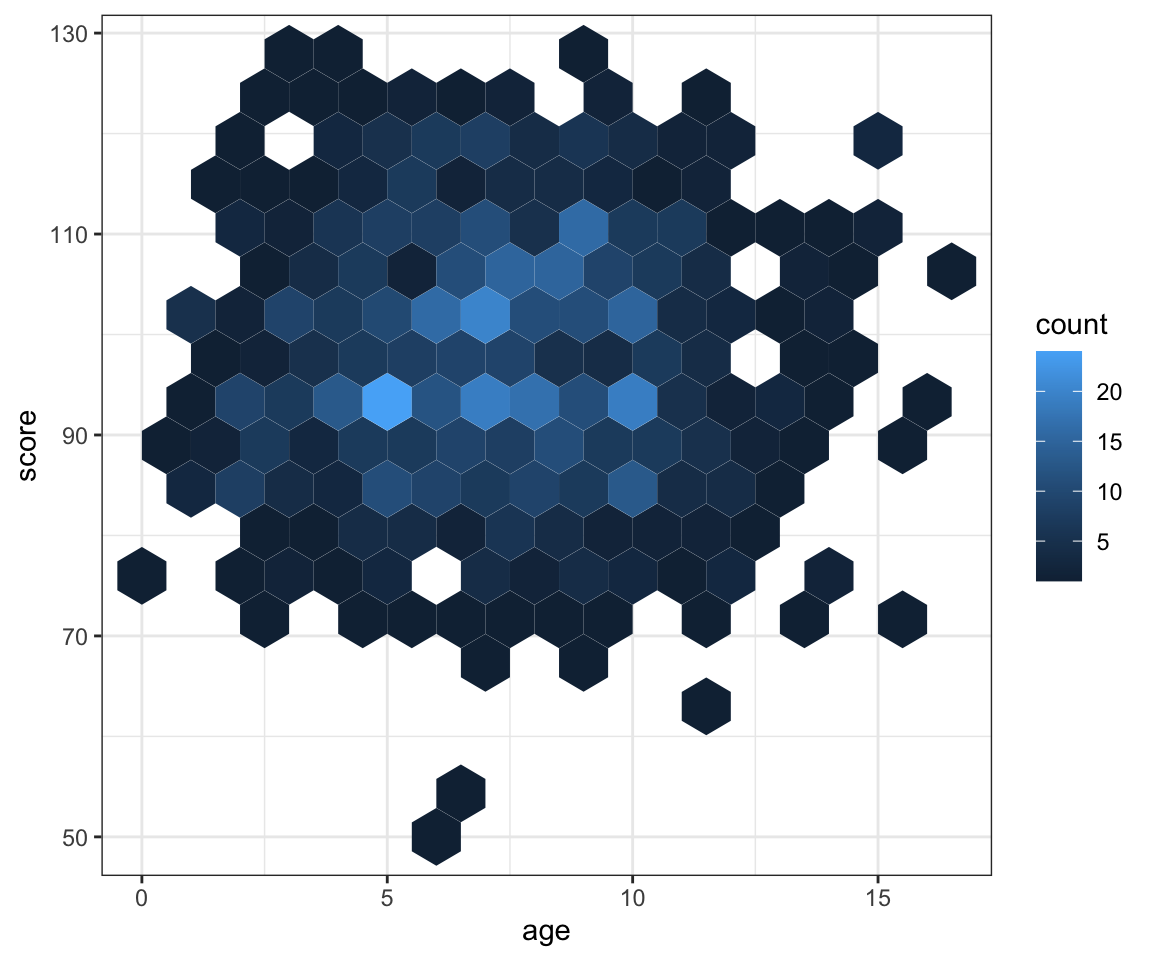Figure 3.27: Hexagonal heatmap of bin counts

### 3.9.4 Correlation Heatmap

I've included the code for creating a correlation matrix from a table of variables, but you don't need to understand how this is done yet. We'll cover `mutate()` and `gather()` functions in the dplyr and tidyr lessons.

``````# generate two sets of correlated variables (a and b)
heatmap <- tibble(
a1 = rnorm(100),
b1 = rnorm(100)
) %>%
mutate(
a2 = a1 + rnorm(100),
a3 = a1 + rnorm(100),
a4 = a1 + rnorm(100),
b2 = b1 + rnorm(100),
b3 = b1 + rnorm(100),
b4 = b1 + rnorm(100)
) %>%
cor() %>% # create the correlation matrix
as.data.frame() %>% # make it a data frame
rownames_to_column(var = "V1") %>% # set rownames as V1
gather("V2", "r", a1:b4) # wide to long (V2)``````

Once you have a correlation matrix in the correct (long) format, it's easy to make a heatmap using `geom_tile()`.

``````ggplot(heatmap, aes(V1, V2, fill=r)) +
geom_tile() +
scale_fill_viridis_c()``````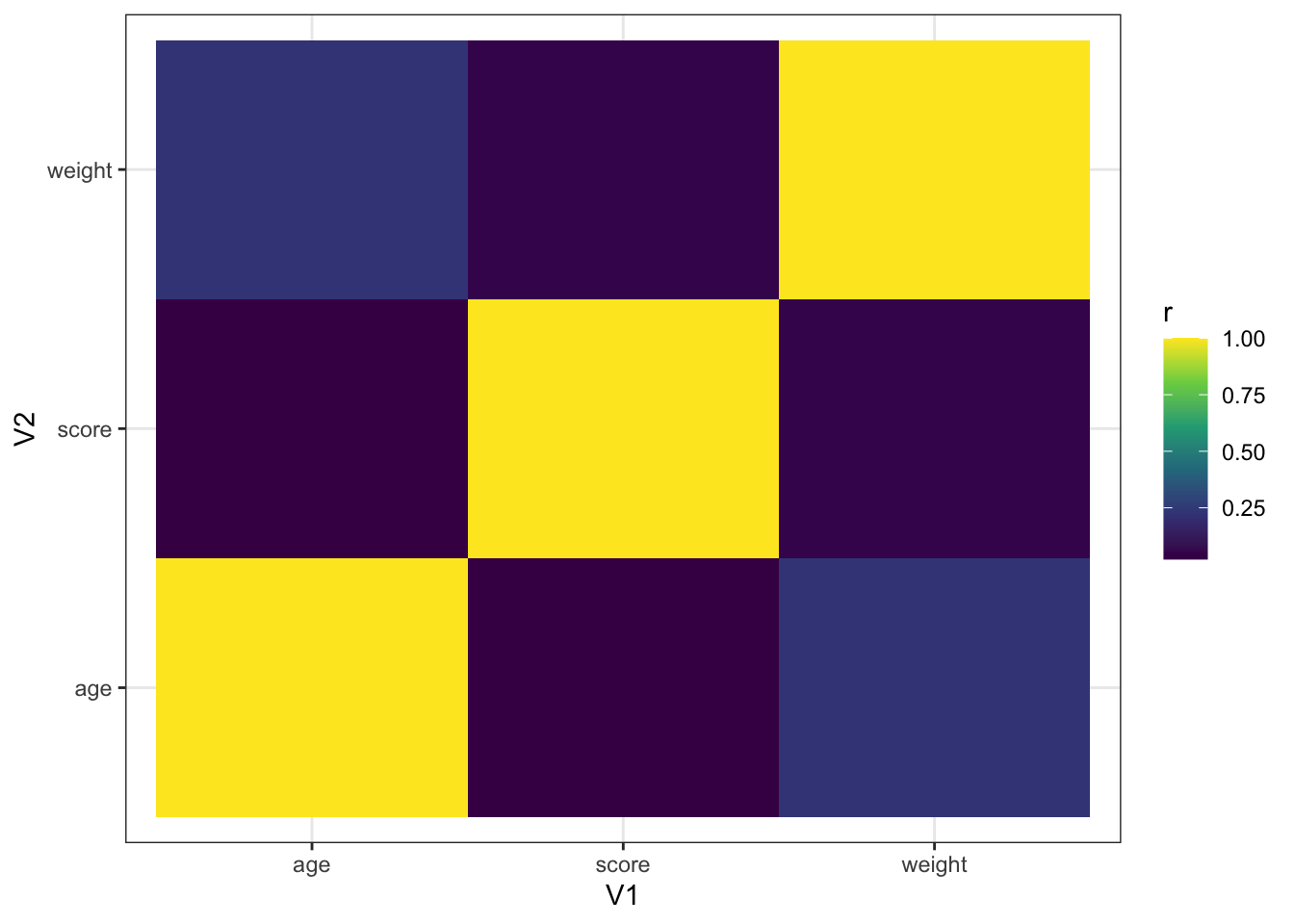Figure 3.28: Heatmap using geom_tile()

The file type is set from the filename suffix, or by specifying the argument `device`, which can take the following values: "eps", "ps", "tex", "pdf", "jpeg", "tiff", "png", "bmp", "svg" or "wmf".

## 3.10 Interactive Plots

You can use the `plotly` package to make interactive graphs. Just assign your ggplot to a variable and use the function `ggplotly()`.

``````demog_plot <- ggplot(pet_happy, aes(pet, happiness, fill=pet)) +
geom_point(position = position_jitter(width= 0.2, height = 0), size = 2)

ggplotly(demog_plot)``````

Figure 3.29: Interactive graph using plotly

Hover over the data points above and click on the legend items.

## 3.11 Quiz

1. Generate a plot like this from the built-in dataset `iris`. Make sure to include the custom axis labels.``````ggplot(iris, aes(Species, Petal.Width, fill = Species)) +
geom_boxplot(show.legend = FALSE) +
xlab("Flower Species") +
ylab("Petal Width (in cm)")

# there are many ways to do things, the code below is also correct
ggplot(iris) +
geom_boxplot(aes(Species, Petal.Width, fill = Species), show.legend = FALSE) +
labs(x = "Flower Species",
y = "Petal Width (in cm)")``````
2. You have just created a plot using the following code. How do you save it?

``````ggplot(cars, aes(speed, dist)) +
geom_point() +
geom_smooth(method = lm)``````
`  ggsave() ggsave("figname") ggsave("figname.png") ggsave("figname.png", plot = cars)`
3. Debug the following code.

``````ggplot(iris) +
geom_point(aes(Petal.Width, Petal.Length, colour = Species)) +
geom_smooth(method = lm) +
facet_grid(Species)``````
``````ggplot(iris, aes(Petal.Width, Petal.Length, colour = Species)) +
geom_point() +
geom_smooth(method = lm) +
facet_grid(~Species)``````4. Generate a plot like this from the built-in dataset `ChickWeight`.``````ggplot(ChickWeight, aes(weight, Time)) +
geom_hex(binwidth = c(10, 1)) +
scale_fill_viridis_c()``````
5. Generate a plot like this from the built-in dataset `iris`.``````pw <- ggplot(iris, aes(Petal.Width, color = Species)) +
geom_density() +
xlab("Petal Width (in cm)")

pl <- ggplot(iris, aes(Petal.Length, color = Species)) +
geom_density() +
xlab("Petal Length (in cm)") +
coord_flip()

pw_pl <- ggplot(iris, aes(Petal.Width, Petal.Length, color = Species)) +
geom_point() +
geom_smooth(method = lm) +
xlab("Petal Width (in cm)") +
ylab("Petal Length (in cm)")

cowplot::plot_grid(
pw, pl, pw_pl,
labels = c("A", "B", "C"),
nrow = 3
)``````

## 3.12 Exercises

Download the exercises. See the plots to see what your plots should look like (this doesn't contain the answer code). See the answers only after you've attempted all the questions.